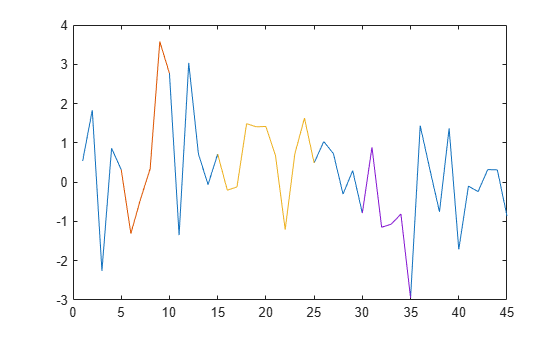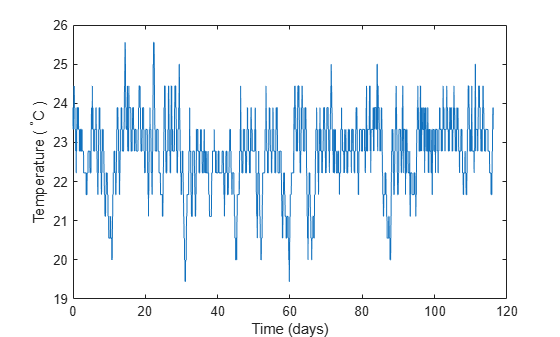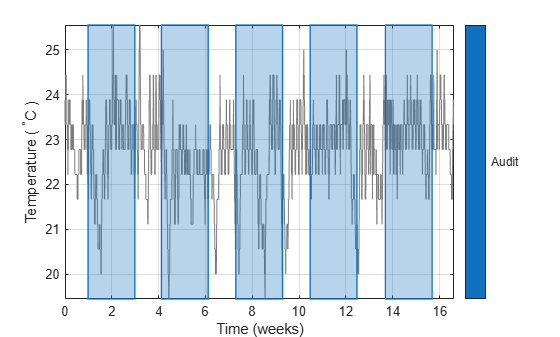extractsigroi

Extract signal regions of interest

Description

example

sigroi = extractsigroi(x,roilims) extracts regions of interest (ROIs) of the input signal vector x based on the ROI limits specified in roilims.

example

sigroi = extractsigroi(x,roilims,concat) with concat specified as true extracts regions of interest and concatenates them.

Examples

collapse all

Consider a two-column matrix representing possible regions of interest of a 45-sample random signal. Extract the signal samples corresponding to the regions of interest.

x = randn(45,1);

roilims = [5 10; 15 25; 30 35];

sigroi = extractsigroi(x,roilims);

Plot the signal and highlight the regions of interest.

plot(x)

hold on
for kj = 1:length(sigroi)
plot(roilims(kj,1):roilims(kj,2),sigroi{kj})
end
hold offConsider a set of temperature data collected by a thermometer inside an office building for about four months. The device takes a reading every half hour. The sample rate is thus 48 measurements/day. Convert the temperature to degrees Celsius and plot the data.

tempC = (temp-32)*5/9;

fs = 48;
t = (0:length(tempC) - 1)/fs;

plot(t,tempC)
xlabel('Time (days)')
ylabel('Temperature ( {}^\circC )')Create region-of-interest limits that separate the temperature data into 29-day periods.

roilims = [1 29; 30 58; 59 87; 88 116];

Extract the regions of interest. Compute the mean temperature of each period and display the values.

sigroi = extractsigroi(tempC,roilims*fs);

cellfun(@mean,sigroi)'
ans = 1×4

22.8819   22.3073   22.7633   23.0066

Consider a two-column matrix representing possible regions of interest of a 45-sample random signal. Extract the signal samples corresponding to the regions of interest. Concatenate the samples into a single vector.

x = randn(45,1);

roilims = [5 10; 15 25; 30 35];

sigroi = extractsigroi(x,roilims,true);

Plot the signal and highlight the regions of interest.

plot(x)

y = NaN(size(x));

for kj = 1:size(roilims,1)
roi = roilims(kj,1):roilims(kj,2);
y(roi) = sigroi(1:length(roi));
sigroi(1:length(roi)) = [];
end

hold on
plot(y)
hold offConsider a set of temperature data collected by a thermometer inside an office building for four months. The device takes a reading every half hour. The sample rate is thus 48 measurements/day. Convert the temperature to degrees Celsius.

tempC = (temp-32)*5/9;

fs = 48;

Create region-of-interest (ROI) limits that correspond to five random two-week periods separated by at least 24 hours. Use the temperature readings from these days for an audit.

r = 5;
w = 14*fs;
s = 1*fs;

hq = histcounts(randi(r+1,1,length(tempC)-r*w-(r-1)*s),(1:r+2)-1/2);
t = (1 + (0:r-1)*(w+s) + cumsum(hq(1:r)))';

roilims = [t t+w-1];

Extract the regions of interest. Compute the mean temperature of each audited region of interest and display the values.

sigroi = extractsigroi(tempC,roilims);

cellfun(@mean,sigroi)'
ans = 1×5

22.8075   22.2586   22.4256   22.9018   23.1457

Extract the regions of interest again, but now concatenate the samples into a single vector. Compute the mean temperature across the audited regions.

sigroic = extractsigroi(tempC,roilims,true);

avgTFc = mean(sigroic)
avgTFc = 22.7078

Convert the ROI limits to a binary sequence and create a mask. Express time in weeks.

Plot the data and visualize the regions of interest with rectangular patches.

plotsigroi(msk,tempC,true)
xlabel('Time (weeks)')
ylabel('Temperature ( {}^\circC )')Input Arguments

collapse all

Input signal, specified as a vector.

Example: chirp(0:1/1e3:1,25,1,50) specifies a chirp sampled at 1 kHz.

Data Types: single | double
Complex Number Support: Yes

Region-of-interest limits, specified as a two-column matrix of positive integers. The ith row of roilims contains nondecreasing indices corresponding to the beginning and end samples of the ith region of interest of a signal.

Example: [5 8; 12 20; 18 25] specifies a two-column region-of-interest matrix with three regions.

Data Types: single | double | int8 | int16 | int32 | int64 | uint8 | uint16 | uint32 | uint64

Option to concatenate extracted signal regions, specified as a logical value.

Data Types: logical

Output Arguments

collapse all

Signal regions of interest, returned as a cell array or a vector.

• If concat is set to false, sigroi is a cell array. The ith cell of sigroi contains the signal samples corresponding to the ith region of interest specified in roilims.

• If concat is set to true, sigroi is a vector that concatenates all extracted signal samples.